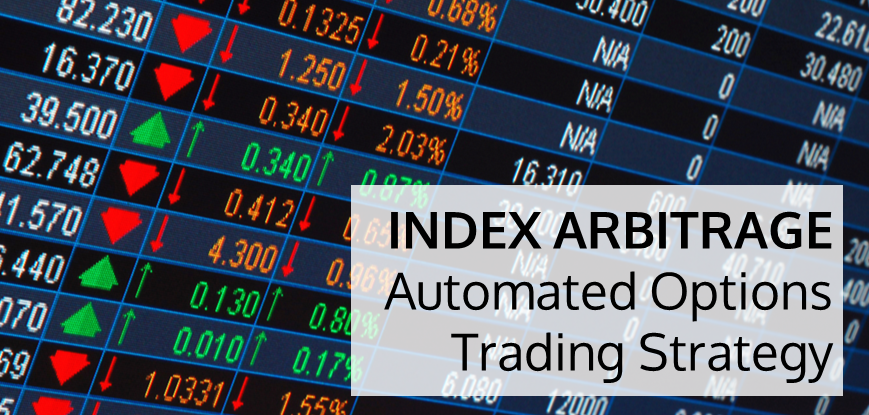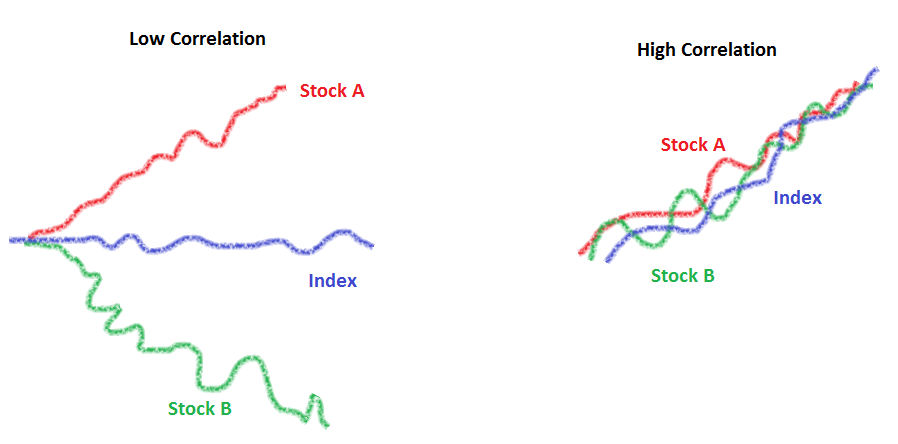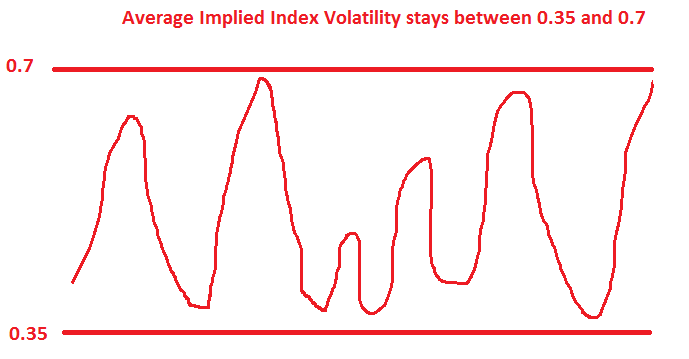# Index Arbitrage - An Automated Options Trading StrategyIn this post, we will discuss automated arbitrage trading (index arbitrage) and the complexities around implementation of this idea without automation. If you are new to learning options trading then you can check the options trading for beginners free course on Quantra.

An index consists of a basket of stocks. Price of the index depends on the prices of the constituent stocks. If the price of all stocks goes by 5%, then the index price also goes by 5%.

Do you think the same will be in case of volatility: If volatility of all stocks goes up by 5%, would the index volatility also increase by 5%? The answer is ‘Not necessarily’! Index volatility depends on the correlation between the stocks. Let us see how.

In the case of low correlation between stocks A and B, even though the volatility of stock A and B are both increasing, the index volatility remains more or less the same. On the other hand, when correlation is high then all the elements of the index are moving together so that volatility of index is high when the volatility of the stocks is high.### Options on Stocks and Index

Volatility used to price index options depends on the volatility used to price the stock options and the correlation between the stocks.

Volatility used to price index options = function (volatility used to price the stock options, correlation level among the stocks)

Based on the price of the index options in the market, we can find the implied volatility used to price the index options.

Similarly, based on the price of the stock options in the market, we can find the implied volatility used to price the stock options.

By plugging in these two values in the function above, average implied level of correlation among the stocks is found.

### Trade Our View on the average implied level of correlation

If say your current correlation is 0.4, you expect the correlation to go up. That means all stocks will move together so index volatility will go up relatively. Similarly if the correlation is expected to go down, then index volatility is expected to go down relative to stock options.When correlation is expected to go up => Volatility of index option is expected to go up => Buy index options & Sell stock options in the ratio of their weightage.

### Why should we go for automated arbitrage trading?

1. Approximation of the index basket

Say, Basket has stock A (43% weightage) and stock B (34% weightage). You approximate it to 44 and 33 so that ratio now becomes 4/3. However, in reality the there are many stocks in an index. What approximation gives you the smallest sizes of stocks with smallest error? On top of it, the lot sizes might be different. This involves complex mathematics that require automation.

1. Calculating total portfolio Greeks by combining Greeks for individual stocks and index.

For example, when you are trading on index Nifty basket, your portfolio has a specific Delta, Vega and Gamma for Nifty and consistent stocks such as SBI, Reliance, TCS, etc. For the net portfolio the Greeks delta, vega and gamma need to be calculated. This needs to be done frequently in real time.

1. As market moves, the position has to be rolled from one set of option strikes to another.

To get a more thorough understanding of automated arbitrage trading you can go through the recording of this discussion here,